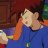# Can anybody help to explain these scripts?

F

#### firefox

##### New member
Hi,

I am a newbie and having difficulty in understanding these TOS lines to determine the peaks. Would appreciate if anybody can help to explain what these lines do and is h1 refers to previous peak and h2 refers to current peak?

def h1 = Highest(high, 20);
def h2 = Highest(high, 15);
def os1=GetMaxValueOffset(high, 20);
def os2=GetMaxValueOffset(high, 15);#### horserider

##### Well-known member
VIP
Maybe this will help.

The indexing operator [] is used to access the previous or future data in an array of data. The square brackets indicate the shift against the current moment. The positive values of the shift represent the values in the past, the negative shift values represent the values in the future. The indexing operator can be applied to fundamental data, function outputs, indicator outputs, variables, etc.

Example 1
input price = close;
input shift = 5;
plot PastPrice = price[shift];
plot FuturePrice = price[-shift];

Draws shifted price plots.

Example 2
plot PastMACD = MACD().Avg;

Shows the smoothed MACD value 10 bars ago.

Last edited by a moderator:
•BenTen
F

#### firefox

##### New member
Thank you @horserider for your quick reply. So does it mean :

def h1 = Highest(high, 20);
Is referring to the highest price 15 bare ago for the last 20 bars

and

def h2 = Highest(high, 15);
refers to the current highest price for the last 15 bars.

Is my understanding correct?

Last edited by a moderator:#### horserider

##### Well-known member
VIP
Highest high of past 20 bars shifted 15 bars

Confusing unless you are a math wizard. Plot h1 and h2 and look at what it does.
plot h1 = Highest(high, 20);
plot h2 = Highest(high, 15);

F

#### firefox

##### New member
Highest high of past 20 bars shifted 15 bars

Confusing unless you are a math wizard. Plot h1 and h2 and look at what it does.
plot h1 = Highest(high, 20);
plot h2 = Highest(high, 15);
Thank you so much. You gave me a great idea to use plot function to View what it does. I will go and try it. Thanks again for solving my problem.Does anybody use gravity points? Questions 3